# Hex, Bugs and More Physics | Emre S. Tasci

a blog about physics, computation, computational physics and materials…

## (7,4) Hamming Code

### November 23, 2007 Posted by Emre S. Tasci

The (7,4) Hamming Code is a system that is used for sending bits of block length 4 (16 symbols) with redundancy resulting in transmission of blocks of 7 bits length. The first 4 bits of the transmitted information contains the original source bits and the last three contains the parity-check bits for {1,2,3}, {2,3,4} and {1,3,4} bits.

It has a transmission rate of 4/7 ~ 0.57 . Designate the source block as s, the transmission operator as G so that transmitted block t is t = GTs and parity check operator is designated as H where: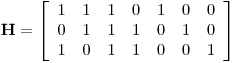This could be more obvious if we investigate the GT and keeping in mind that, the first 4 "transformed" bits stay the same with 3 added to the end for parity check. So our parity reporter operator P would be: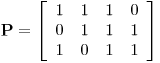As it calculates the parities for bits, also its Modulo wrt 2 must be included. Since we’re calculating the parities for {1,2,3}, {2,3,4} and {1,3,4} bit-groups, the values of the P are easily understandable. Now, we will join the Identity Matrix of order 4 (for directly copying the first 4 values) with the P operator to construct the GT transformation (encoder) operator:and H is actually nothing but [-P I3]. It can be seen that HGTs will always result {0,0,0} in a parity check, meaning that all three parities have passed the test. So, where is the problem?

Noise: Noise is the operator that flips the values of bits randomly with an occuring probability f. Suppose that we have a source signal block of 4 bits length: {s1, s2, s3, s4}. I encode this information with calculating the parities and adding them to the end, thus coming up with a block of 7 bits length and call this block as t (for transmitted):

t=GTs (supposing t and s are given as column vectors. Otherwise, t=sG)

Now I send this packet but on the road to the receiver, the noise changes it and so, the received message, r, is the transmitted message (t) AND the noise (n):

r=t+s

Since I have got the parity information part (which may also have been affected by the noise), I compare the received data’s first 4 values with the parity information stored in the last 3 values. A very practical way of doing this is using 3 intersecting circles and placing them as: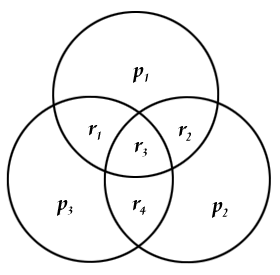with r1-r4 representing the first values (ie, actual data) received and p1-p3 being the parity information (the last 3 values). So we place the values to their corresponding locations and easily can check if the parity matches to the related values. We can have 8 different situations at this moment. Let’s denote the z=Hr vector as the parity check result. z={0,0,0} if all three parity information agrees with the calculated ones, z={1,0,0} if only p1 is different from the calculated parity of {r1,r2,r3}, z={1,0,1} if p1 AND p3 are different from their corresponding calculated parities and so on. If there is only 1 error (ie, only one "1" and two "0") in the parity check, then it means that, the noise affected only the parity information, not the data encoded (ie, the first 4 values), so the corrupted data can be fixed by simply switching the faulty parity. If there are 2 parity check errors, this time, the value on the intersection of the corresponding parities (ie, r1 for p1&p3, r2 for p1&p2 and r4 for p2&p3,) must be switched. If z={1,1,1} then r3 should be switched.

Let’s introduce some mathematica operations and then tackle with some examples. You can just copy and paste them since there are no additional libraries required:

mGT = {{1,0,0,0},{0,1,0,0},{0,0,1,0},{0,0,0,1},{1,1,1,0},{0,1,1,1},{1,0,1,1}};
mH = {{1,1,1,0,1,0,0},{0,1,1,1,0,1,0},{1,0,1,1,0,0,1}};

(mS = {{1},{1},{1},{0}});
Mod[(mGTS=mGT . mS),2] // MatrixForm
mN ={0,0,0,0,0,0,1};
(mR = Mod[mGTS+mN,2])  // MatrixForm
mZ = Mod[mH . mR,2];
Flatten[mZ]

The first two lines define GT and H and from here, you can check a very important property being:

Mod[mH.mGT,2] // MatrixForm

equal to 0.

We introduce the 4bit data to be transmitted as mS which we had referred as s through out the text. Then we encode the data to be send with the parity info encoded as mGTS (t) add a noise mN (n) to it assuming it is corrupted in the transmission process and the data the other side receives is mR (r) consisting of the data transmitted AND the noise acted on it. mZ is the parity check result (z) (I’ve "Flatten"ed it for a better view). In the operations above, the "Mod" operator is frequently used because of our universe being a mod2 universe (2=0, 3=1, 4=0,… just 0s and 1s actually!).

So, in this example, we had s={1,1,1,0}, and this was encoded as t={1,1,1,0,1,0,0} and on its way to the happy-ending, a noise corrupted it and switched its 3rd parity information from 0 to 1. As a result of this, the recipient got its data as r={1,1,1,0,1,0,1}. But since our recipient is not that dull, a parity check is immediately applied and it was seen that z={0,0,1} meaning that, there is something wrong with the 3rd parity information, and nothing wrong with the information received at all!

Now, let’s apply all the possible noises given that only one value can be switched at a time (ie, only one 1 in the n vector with the remaining all being 0s).

For[i = 1, i <= 7, i++,
mN = {0, 0, 0, 0, 0, 0, 0} ;
mN = ReplacePart[mN, 1, i];
mR = Mod[mGTS + mN, 2];
mZ = Mod[mH . mR, 2];
Print[mN, "\t", Flatten[mZ]];
];

After this, if everything went right, you’ll see the output something like this:

{1,0,0,0,0,0,0}   {1,0,1}
{0,1,0,0,0,0,0}   {1,1,0}
{0,0,1,0,0,0,0}   {1,1,1}
{0,0,0,1,0,0,0}   {0,1,1}
{0,0,0,0,1,0,0}   {1,0,0}
{0,0,0,0,0,1,0}   {0,1,0}
{0,0,0,0,0,0,1}   {0,0,1}

So we know exactly what went wrong at are able to correct it… Everything seems so perfect, so there must be a catch…

There is no decoding error as long as there is only 1 corruption per data block!

As the corruption number increases, there is no way of achieving the original data send with precise accuracy. Suppose that we re-send our previous signal s={1,1,1,0} which yields t={1,1,1,0,1,0,0} but this time the noise associated with the signal is n={0,1,0,0,0,0,1} so, the received signal is r={1,0,1,0,1,0,1}. When we apply the partition check, we have z={1,1,1}, which means that, there is a problem with the 3rd value and we deduce that the original message transmitted was t={1,0,0,0,1,0,1} which passes the parity check tests with success alas a wrongly decoded signal!

The probability of block error, pB is the probability that there will at least be one decoded bit that will not match the transmitted bit. A little attention must be paid about this statement. It emphasizes the decoded bit part, not the received bit. So, in our case of (4,7) Hamming Code, there won’t be a block error as long as there is only one corrupted bit in the received bit because we are able to construct the transmitted bits with certainty in that case. Only if we have two or more bits corrupted, we won’t be able to revive the original signal, so, pB scales as O(f2).

References : I have heavily benefitted from David MacKay’s resourceful book Information Theory, Inference, and Learning Algorithms which is available for free for viewing on screen.Information Theory, Inference, and Learning Algorithms by David MacKay

## A Crash Course on Information Gain & some other DataMining terms and How To Get There

### November 21, 2007 Posted by Emre S. Tasci

Suppose that you have -let’s say- 5 pipelines outputting Boolean values seemingly randomly such as: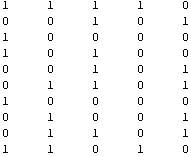Now, we will be looking for a possible relation between these variables -let’s name that {a,b,c,d,e}ï¿½ (Suppose that we do not know that the first 3 variables (a,b,c)ï¿½ are randomly tossed, while the 4th (d)ï¿½ is just “a AND b” and the 5th one (e) is actually “NOT a”).

1. We look for different possible values of each variable and store them in an array called posVals (as obvius, from “Possible Values”). In our example, each of the variables can have only two values, so our posVals array will look something like this: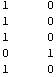2. Now that we know the possible outcomes for each of the variables, we calculate the probability of each of these values by simply counting them and then dividing to the total number of output. As an example, for the 3rd variable,ï¿½ there are four 0’s and 6 1’s totaling 10 values. So, the probability of a chosen value to be 0 would be 4 out of 10 and 6/10 for 1. We store the probabilities in an array called probs this time which is like
ï¿½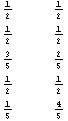In theï¿½ 3rd row, the probabilities for the c variable are stored in the order presented in the posVals array (check the 3rd row of posVals to see that the first value is 1 and the second is 0). You can object the unnecessity of storing the second row of probs because, the second row is always equal to 1-probs[] (I will be using the Mathematica notation for array elements, with a[] meaning the 1st row if a is a matrix, or 1st element if a is a 1-D List; a[][] meaning the element on the first row and third column of the matrix a), but it is only a specific case that we are dealing with Boolean outputs. Yes,ï¿½ a variable’s probability always equals to the sum of the probabilities of all the other variables subtracted from 1 but we will keep record of all the probabilities for practical reasons.
3. Now we will calculate the entropy for each variable. Entropy is a measure of order in a distribution and a higher entropy corresponds to a more disordered system. For our means, we will calculate the entropy as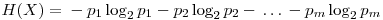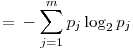In these formulas, H represents the entropy,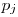represents the probability of the jth value for variable X. Let’s store this values in the entropies array.
4. Special Conditional Entropy,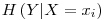ï¿½ is defined as the entropy of the Y variable values for which the corresponding X variable values equal to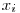.ï¿½ Forï¿½ our given example, let’s calculate H(d|a=1): Searching through the data, we see that, a=1 for rows: {1,3,4,7,10} and the values of d in these rows correspond to: {1,0,0,0,1}. Let’s assume this set ({1,0,0,0,1}) as a brand new set and go on from here.ï¿½ Applying the definition of the entropy given in the 3rd step, we have: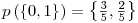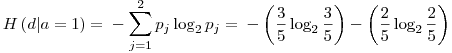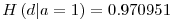5. Conditional Entropy,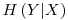is the entropy including all the possible values of the variable being tested (X)ï¿½ as having a relation to theï¿½ targetedï¿½ variable (Y). It is calculated as: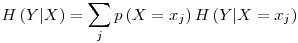Again, if we look at our example, since we have H(d|a=1) =ï¿½ 0.970951, p(a=1) = 0.5 ; and if we calculate H(d|a=0) in a similar way as in step 4, we find H(d|a=0) = 0 and taking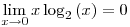we find that H(d|a) = 0.5 x 0.970951 + 0.5 x 0 = 0.4854755
6. Information Gain,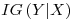– is a measure of the effect a variable (X)ï¿½ is having againstï¿½ a targetted variable (Y) with the information on the entropy of the targetted variable itself (H(Y))ï¿½ taken into account. It is calculated as: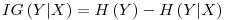For our example , H(d) = 0.721928 and we calculated H(d|a=0) = 0.4854755 so, IG(d|a) = 0.236453. Summing up, for the data (of 10 for each variable)ï¿½ presented in step 1, we have the Information Gain Table as:

 X IG(e|X) X IG(d|X) X IG(c|X) a 1.0 a 0.236453 a 0.124511 b 0.0290494 b 0.236453 b 0.0 c 0.124511 c 0.00740339 d 0.00740339 d 0.236453 e 0.236453 e 0.124511
 X IG(b|X) X IG(a|X) a 0.0290494 b 0.0290494 c 0.0 c 0.124511 d 0.236453 d 0.236453 e 0.0290494 e 1.0

And here are the results for 1000 values per variable instead of 10:

 X IG(e|X) X IG(d|X) X IG(c|X) a 0.996257 a 0.327922 a 0.000500799 b 0.000716169 b 0.29628 b 0.00224924 c 0.000500799 c 0.000982332 d 0.000982332 d 0.327922 e 0.327922 e 0.000500799
 X IG(b|X) X IG(a|X) a 0.000716169 b 0.000716169 c 0.00224924 c 0.000500799 d 0.29628 d 0.327922 e 0.000716169 e 0.996257

What can we say about the relationships from these Information Gain results?

• H(Y|X) = H(X|Y) – Is this valid for this specific case or is it valid forï¿½ general?
• Variable a: We can most probably guess a if we had known e, and it is somehow correlated with d.
• Variable b: It is correlated with d.
• Variable c: There doesn’t seem to be any correlation.
• Variable d: It is equally correlated with a&e and also correlated with b very closely to that correlation with a&e.
• Variable e: It is VERY strongly correlated with a andï¿½ it is somehow correlated with d.

Let’s take it on from here, with the variable d:

This is the IG table for 10000 data per each variable:

 X IG(d|X) a 0.30458 b 0.297394 c 0.00014876 e 0.30458

This is the IG table for 100000 data per each variable:

 X IG(d|X) a 0.309947 b 0.309937 c 1.97347×10^-6 e 0.309947

You can download the Mathematica sheet for this example : Information Gain Example

You will also need the two libraries (Matrix & DataMining) to run the example.

## Mathematica Matrix Examples

### November 19, 2007 Posted by Emre S. Tasci

Mathematica Matrix Manipulation Examples

B={Range,Range[6,10],Range[11,15],Range[16,20]} ;
TableForm[B]

(* To extract rows 2 & 3 *)
Part[B,{2,3}] // TableForm

(* To extract cols 2 & 4 *)
Part[B, All,{2,4}] // TableForm

(* To extract the intersection of Rows 2&3 with Cols 2&4 *)
Part[B,{2,3} ,{2,4}] // TableForm

(* Selecting the values from the 2. row where B[][[i]] has a specific value like <10 *)
Select[B[],#<10&]

(* Selecting the values from the 2. col where B[[i]][] has a specific value like <10 *)Select[Part[B,All,2],#<10&]

(* Alternative Transpose (//Equivalent to Transpose[matrixIN]//)*)
TransposeEST[matrixIN_]:=Module[
{matrixOUT},
numCols = Dimensions[matrixIN][];
matrixOUT = Array[mo,numCols];
For[i=1,i<=numCols,i++,
ï¿½ ï¿½ ï¿½ matrixOUT[[i]]=Part[matrixIN,All,i];
]
TableForm[matrixOUT];
Return[matrixOUT];
]

(* Swapping two rows *)
({B[],B[]}={B[],B[]}) // TableForm

(*Reading data from a file with a content like this:
0.642864000  0.761961000  1.665420000
5.815150000  3.603020000  1.831920000
6.409050000  7.150810000  0.966674000
5.389080000  4.989350000  6.571080000
6.044070000  4.887170000  7.005700000
6.806280000  2.766540000  4.030720000
6.676540000  0.652834000  5.729050000
1.231490000  5.754580000  0.404114000
9.722660000  7.571420000  1.133980000
8.869320000  7.574570000  4.886290000
1.221750000  8.511380000  6.201370000
2.817450000  0.216682000  6.474590000
5.638430000  7.422500000  7.381840000
8.903770000  4.192770000  0.355182000
7.073810000  1.421990000  1.606240000
7.646660000  1.979770000  3.877830000
8.239190000  1.280060000  6.705840000
4.430740000  2.016050000  5.515660000
9.771000000  2.637290000  8.423900000
8.916410000  4.900040000  2.592560000

*)

Close[m];
(k =Partition[k,3]) // TableForm

(* And you can definitely Plot it too *)
<<GraphicsGraphics3D
ScatterPlot3D[k,PlotStyle®{{PointSize[0.04],GrayLevel[0.09]}}]

## A Mathematica Example for Information Gain

### Posted by Emre S. Tasci

Mathematica Code for Andrew Moore – Information Gain Example This is what I’ve written implementing the two libraries. It calculates the information gain for Andrew Moore’s example in his Information Gain example (which can be accessed from his resourceful website on datamining: http://www.autonlab.org/tutorials/)

You will be needing the two libraries and you can download them from the related entries for Matrix and DataMining procedures.

## DataMining Functions Library for Mathematica

### Posted by Emre S. Tasci

Mathematica Library for DataMining
Functions Library Intended To Be Used InÂ DataMining Procedures
11/2007
Emre S. Tasci
S&C / MSE / 3mE / TUDelftFunctions List (EF: Required External Functions)

• Int DMBooleEST[statement_]
Converts True/False => 1/0
EF – NONE
• Bool DMBooleEST[statement_]
Converts 1/0 => True/False
EF – NONE
• Int DMCondEntropyEST[MatrixIN_,var2_,var1_, posVal1_, posVal2_,probVar1_]
Calculates the Conditional Entropy H(var2|var1)
EF – MSelectEST, DMProbeEST, DMEntropyEST
• Int DMEntropyEST[problist_]
Calculates the entropy given the probability list “problist”
EF – NONE
• Matrix DMGenMatrixEST[dim_,file_]
Generates a Matrix of [dim,dim] dimension and writes this matrix to the “file” file. Returns the matrix generated.
EF – DMBooleEST, DMUnitizeEST
• List DMInfGainEST[MatrixIN_,varno_]
Calculates the Information Gain related to the “varno” variable wrt to the remaining variables.
EF -Â MDiffElementEST , MSwapEST, DMCondEntropyEST, DMEntropyEST, DMProbeEST
• Vector DMProbeEST[MatrixIN_,varno_,varlist_]
Calculates the probabilities of the variables’ possible outcomes.
EF – NONE;

Important!!! ————————————

Assumed form of the MatrixIN: ;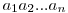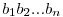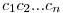(i.e., values for the same variable are stored in ROWS, NOTÂ in COLUMNS!)